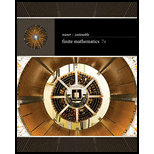# Let p: “Willis is a good teacher,” q: “Carla is a good teacher,” r: “Willis’ students hate math,” and s: “Carla’s students hate math.” Express the statements in Exercises 17–24 in words. ( ∼ s ) ∨ ( ∼ r )### Finite Mathematics

7th Edition
Stefan Waner + 1 other
Publisher: Cengage Learning
ISBN: 9781337280426### Finite Mathematics

7th Edition
Stefan Waner + 1 other
Publisher: Cengage Learning
ISBN: 9781337280426
Chapter A, Problem 22E
Textbook Problem
1 views

## Let p: “Willis is a good teacher,” q: “Carla is a good teacher,” r: “Willis’ students hate math,” and s: “Carla’s students hate math.” Express the statements in Exercises 17–24 in words. ( ∼ s ) ∨ ( ∼ r )

To determine

The meaning of the statement (s)(r) in words, if the statements are,

Statement p: Willis is a good teacher.

Statement q: Carla is a good teacher.

Statement r: Willis’ students hate math.

Statement s: Carla’s students hate math.

### Explanation of Solution

Given Information:

The provided statements are,

Statement p: Willis is a good teacher.

Statement q: Carla is a good teacher.

Statement r: Willis’ students hate math.

Statement s: Carla’s students hate math.

Consider the provided statements,

Statement p says “Willis is a good teacher”.

Statement q says “Carla is a good teacher”.

Statement r says “Willis’ students hate math”.

Statement s says “Carla’s students hate math”.

The not statement of s, that is, s will be “Carla’s students do not hate math”.

The not statement of r, that is, r will be “Willis’ students do not hate math”.

The symbol implies disjunction which means that if either of the two statements is true or both statements are true then the conclusion of the statements will be true

### Still sussing out bartleby?

Check out a sample textbook solution.

See a sample solution

#### The Solution to Your Study Problems

Bartleby provides explanations to thousands of textbook problems written by our experts, many with advanced degrees!

Get Started

Find more solutions based on key concepts
In Exercises 4756, solve the given equation for the indicated variable. 3x212=127

Finite Mathematics and Applied Calculus (MindTap Course List)

A sample with a mean of M = 8 has X = 56. How many scores are in the sample?

Essentials of Statistics for The Behavioral Sciences (MindTap Course List)

Show that an equation of a circle can be written in the form x2 + y2 + Cx + Dy + E = 0 where C, D, and E are co...

Applied Calculus for the Managerial, Life, and Social Sciences: A Brief Approach

Solving an Equation In Exercises 11-26, solve for x. 3=81

Calculus: Early Transcendental Functions

Evaluate the integrals in Problems 7-36. Check your results by differentiation. 7.

Mathematical Applications for the Management, Life, and Social Sciences

Evaluate the integral, if it exists. 0/4(1+tant)3sec2tdt

Single Variable Calculus: Early Transcendentals

Define the concept of “stability” within a phase, and explain why it is important.

Research Methods for the Behavioral Sciences (MindTap Course List)

The graph of f(x) = 3x5 − 5x3 is:

Study Guide for Stewart's Single Variable Calculus: Early Transcendentals, 8th

A parameterization of the curve at the right is:

Study Guide for Stewart's Multivariable Calculus, 8th